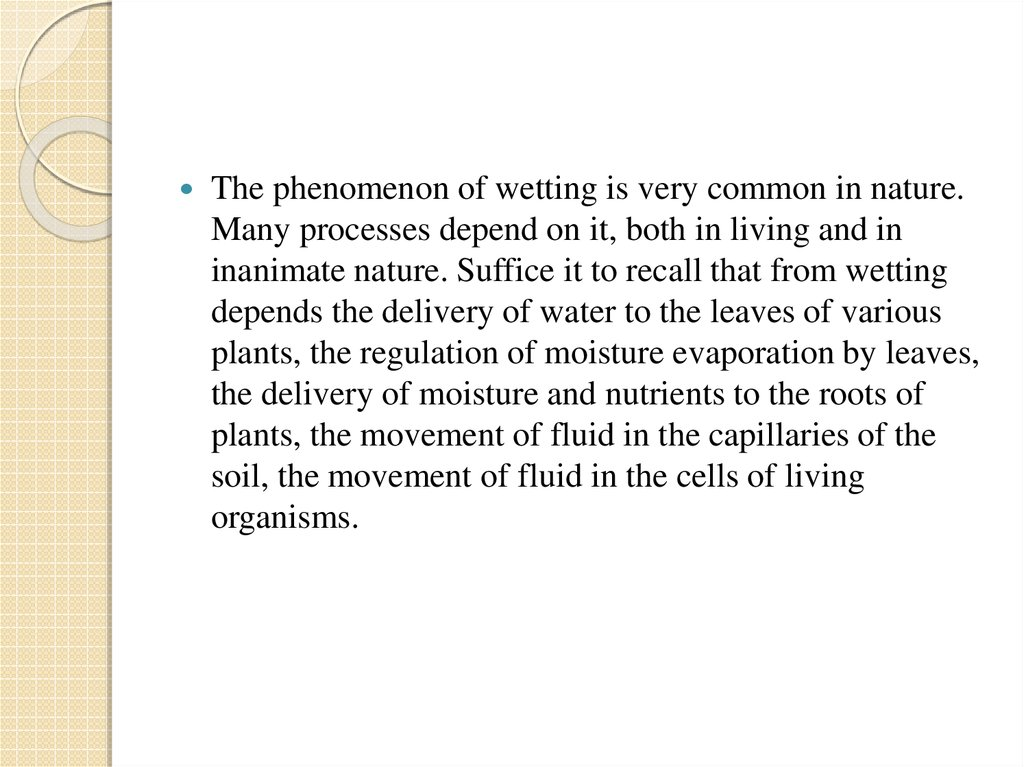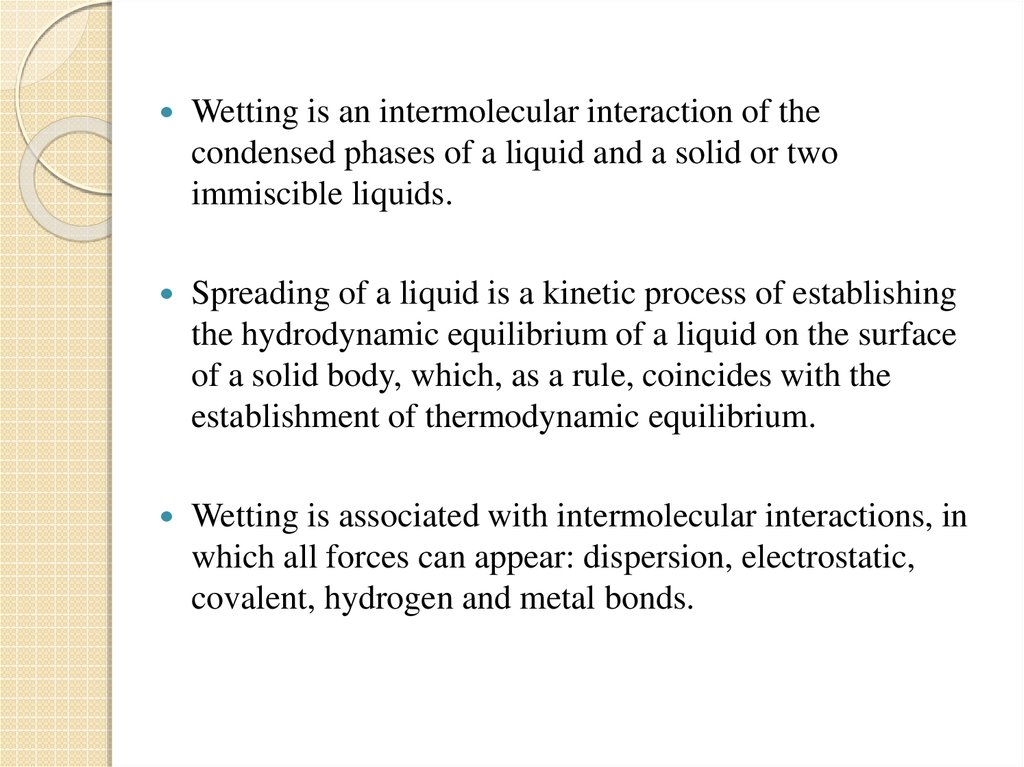Wetting - Thermodynamic conditions

1. Wetting - Thermodynamic conditions

Wetting Thermodynamic
conditions

2.

The phenomenon of wetting is very common in nature.
Many processes depend on it, both in living and in
inanimate nature. Suffice it to recall that from wetting
depends the delivery of water to the leaves of various
plants, the regulation of moisture evaporation by leaves,
the delivery of moisture and nutrients to the roots of
plants, the movement of fluid in the capillaries of the
soil, the movement of fluid in the cells of living
organisms.

3.

Wetting is an intermolecular interaction of the
condensed phases of a liquid and a solid or two
immiscible liquids.
Spreading of a liquid is a kinetic process of establishing
the hydrodynamic equilibrium of a liquid on the surface
of a solid body, which, as a rule, coincides with the
establishment of thermodynamic equilibrium.
Wetting is associated with intermolecular interactions, in
which all forces can appear: dispersion, electrostatic,
covalent, hydrogen and metal bonds.

4.

Consider a drop on a flat substrate. We assume that it
has the shape of a spherical segment and forms an
equilibrium angle θ0 in equilibrium. We introduce the
system from equilibrium by increasing the radius of the
contact spot by Δx. Let us determine the change in the
free energy

5.

We put Δ F equal to zero, since in equilibrium F has a
minimum value. Hence we get the equilibrium value of
the edge angle θ0 :

6. Thermodynamic wetting conditions

It follows from Young's formula if:
• θ0 <900 the angle edge and the liquid wet
the surface; (Non-wetting)
• θ0 >900 the angle is obtuse and the liquid
does not wet the surface (Limited wetting)
• θ0 -900 The angle is not established and the
liquid spreads over the surface (Complete